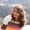FREELessons: 20Length: 1.7 hours• Overview
• Transcript

# 3.4 if and when as Expressions

In this lesson, I'll show you how to use if and when expressions to write neat code in Kotlin. Of course, I'll show you some examples of how this works.

## 5.Conclusion1 lesson, 01:17

### 3.4 if and when as Expressions

Hey there, this lesson onwards, after a few lessons, we will be learning about the Control Statements. And in this lesson, we will be learning about using if and when as expressions in Kotlin. I have written a few lines of code here, as the initial set up of this lesson. What I have done here is, in the main function I have defined two variables num 1 and num 2 which are in data type and have assigned a value of four and five to it. And then in the third variable which is in the third type, I have assigned the greater of the two variables. If num1 is greater than num2, the value of big is equal to num1, else the value of big is num2. And then I have printed the statement which says bigger of $num1 and$num2 is \$big. And I have used the string interpolation here which we had learned few lessons ago. Let's run it, for old times sake, and see what happens. The bigger of four and five is five. So here we have our code up and running. Let us see how do we use if as expression here. To use if as expression, what we will do is var big, which is of integer type, is equal to as num2. What happens here is, if num1 is greater than num2, num1 is written and assigned to big as num2 is returned and assigned to big. Now let us see what happens. And let me change it so that you know it is from the current execution. And here we have bigger of 4 and 5 is 5. So we see we have successfully used if as expression here. Now, what if we have multiple line of code inside the if and the else block. We open and close the curly braces. Same in case of if. Now, let us see what happens. Here since num1 is not greater than num2 it comes to the else block and then the print statement is executed and then num2 is written and assigned to big. So we conclude here that the last statement is written from each of the block. Now let us jump to using when as expression. I'll be removing all this code, because we don't need it any more, just leaving num1 and num2, changing it to side. We have switch case in all other programing languages, like Java, C, C++, but in Kotlin, instead of switch case, when is used. So how do we use when in Kotlin? It has a same syntax as switch, so let us write it. When the comparison variable will be side and since we have multiple line of code, we will open the curly braces and then start writing our cases. Before that, let us define a variable output, which will be off tripe String. Let's start writing the cases When the side doesn't have the value of one, three, or four, what should it do? In the else block, we will have the output as. Now, we will print output. Now let us run our our code and see what happens Since the value of side is full, this condition is satisfied. And the value of output is square, or rectangle may have four sides. String interpolation here again. Instead of having just one condition check, we may have here more than one. Let's say 3 or 6 or 7. The output we will have is, Now let us change the value of side to seven and see what we get. So here we have the value for the output which is it is Triangle, Hexagon, or Septagon. This way we can compare multiple values in a single condition chain in case of when. Now, how is this part executed? Let us change it to 11 and C. So here, we have 11 is not decoded. And hence that part is executed by default. This was the traditional method of using when n. How do we use when as an expression? Let us do it now. That output which is our string type will be equal to, And then, what we do is when side is 1 output is equal to straight line have this much side. When it is 3,6, or 7, this output, is equal to, So here we have used whenn as expression which assigns the shortest string value according to the conditions to that output. Now let us see what happens. Let us change it to one. And here we have, straight line have one side, so we have successfully used when as expression. Now in case if you want to have multiple lines for the conditions,we can put the statements inside the block like this. So here we have two statements inside this block when the value outside is 4. Let us change it to four and see what happens. We see the last value is returned here, but if we want to have multiple line of code, let's say we want to print a statement. Here it says write it like this. Let's see what happens. Here we have, square may have four sides. It is printed and executed from this line. And then, rectangle may have four sides, is written and assigned to output which is then printed here. So this was all about using when and if as expressions. Keep playing around with the code. In the next lessons, we will be learning about loops and iterators in Kotlin. Until then, keep smiling, happy coding, and have a good day.

Back to the top